## 闲聊

c++非常灵活，但是我学习的时候是课本是以 c++98标准的,emm，非常老了。现在使用 c++11甚至c++20 ,感觉98和11以及不是一种语言了，太大的差异。

• Effeetive C++
• More Effeetive C++

• 类 构造析构函数
• 重载以及重载符号
• 友元函数
• 等知识点

1. 比如右值引用的问题
2. 还学习到了一个小技巧，传入参数是类的对象时，函数执行的时候会调用析构函数和拷贝构造函数，如果该函数需要频繁的调用，无疑是增加了时间开销，我们可以传入引用，但是这个函数不具有在修改参数中的对象的功能，比如求两个串的比较，传入静态引用就好

## 认识串

#### 初步了解

==s = "a1a2a3.....an"==

• s成为串名
• n成为串长
• 串长为0的字符串为空串

### 串的基本操作

1. StrAssign(&T,chars); chars是字符常量， 生成一个存储着chars的串T
2. StrCopy(&T,S); 将串S复制到 T中
3. StrEmpty(S);判断 串S是否为空串
4. StrCompare(S,T); 比较S,T字符串
5. StrLenth(S); 获取 S串的长度
6. ClearString(&S); 清空串的长度
7. Concat(&T,S1,S2); 将串拼接到 S中
8. SubString(&Sub,pos,len); 求在pos位置起长度为 len的字串sub
9. Index(S,T,pos);模式匹配，字串T在 主串S中的第pos起，再字串第几个位置
10. Repalce(&S,T,V); 使用串V替换主串中出现和T一样且不重叠的串。
11. StrInsert(&S,pos,T);在第pos位置上插入串 T.
12. StrDlete(&S,pos,len);删除串在pos位置上 长度为len的字串。
13. DestroyString(&S); 销毁串

## 串的实现

### 使用定长的静态顺序表

#define MAXSTRLEN 255
typedef unsiged char SString[MAXSTRLNE + 1]//第0号位置存储着 字符串的长度


unsigned 在c语言就是无符号的(本质上就是 ，原码最高位1不代表是负数 )，这里修饰char，char在c语言占有一个字节，那么 unsiged char 可以表示整型数据的范围就是 0~255(28).

### 使用堆内存存储(动态顺序表)

typedef struct {
char * ch;//如果为空串，ch为NULL
int length;
}HString;


#### 比较

StrCompare(HString S,HString T)

int StringCompare(Hstring S,Hstring T)
{
//如果S>T 则返回 >0 ;如果相等返回 0；如果S<T ,返回 <0
for(i=0;i<S.length&&i<T.length;i++)
if(S.ch[i]!=T.ch[i]) return S.ch[i] -T.ch[i];
return S.lenght-T.lenght;
}


1. S,T等长 但是字符不一样,例如 S=''abb'' T=''abc''
2. S,T不等长 但是存在字串和主串的关系,例如 S=''abbbbb'' T=''abb''
3. S,T 等长 ，字串且一致
4. 等等
for(i=0;i<S.length&&i<T.length;i++)
if(S.ch[i]!=T.ch[i]) return S.ch[i] -T.ch[i];


return S.lenght-T.lenght;


#### 求字串

SubString(HString &Sub,HString S,int pos,int len)

status SubString(HString &Sub,HString S,int pos,int len)
{
if(pos<1||pos>S.length||len<0||len> S.length - pos)
return ERROR;
if(Sub.ch) free(ch); //清空原有串
if(!len) {Sub.ch=NULL;Sub.length=0} //如果len==0
else
{
Sub.ch=(char*)malloc(len*sizeof(char));
Sub.ch[0....len-1]=S.ch[Pos-1....Pos+len-2];
Sub.length=len;
}
return OK;
}


### 块链存储

#define CHUNKSIZE 80 //每一块的大小
typedef struch Chunk
{
char ch[CHunKSize];
struck Chunk * next;
}Chunk;
typedef stuck
{
int curlen;//当前串的长度
}


## 模式匹配

### 基本概念

S 叫 主串，匹配的字串T 叫做模式串，本函数就是求得模式串在主串中的位置，故而叫做 模式匹配

​ 模式串 abcac

### 穷举法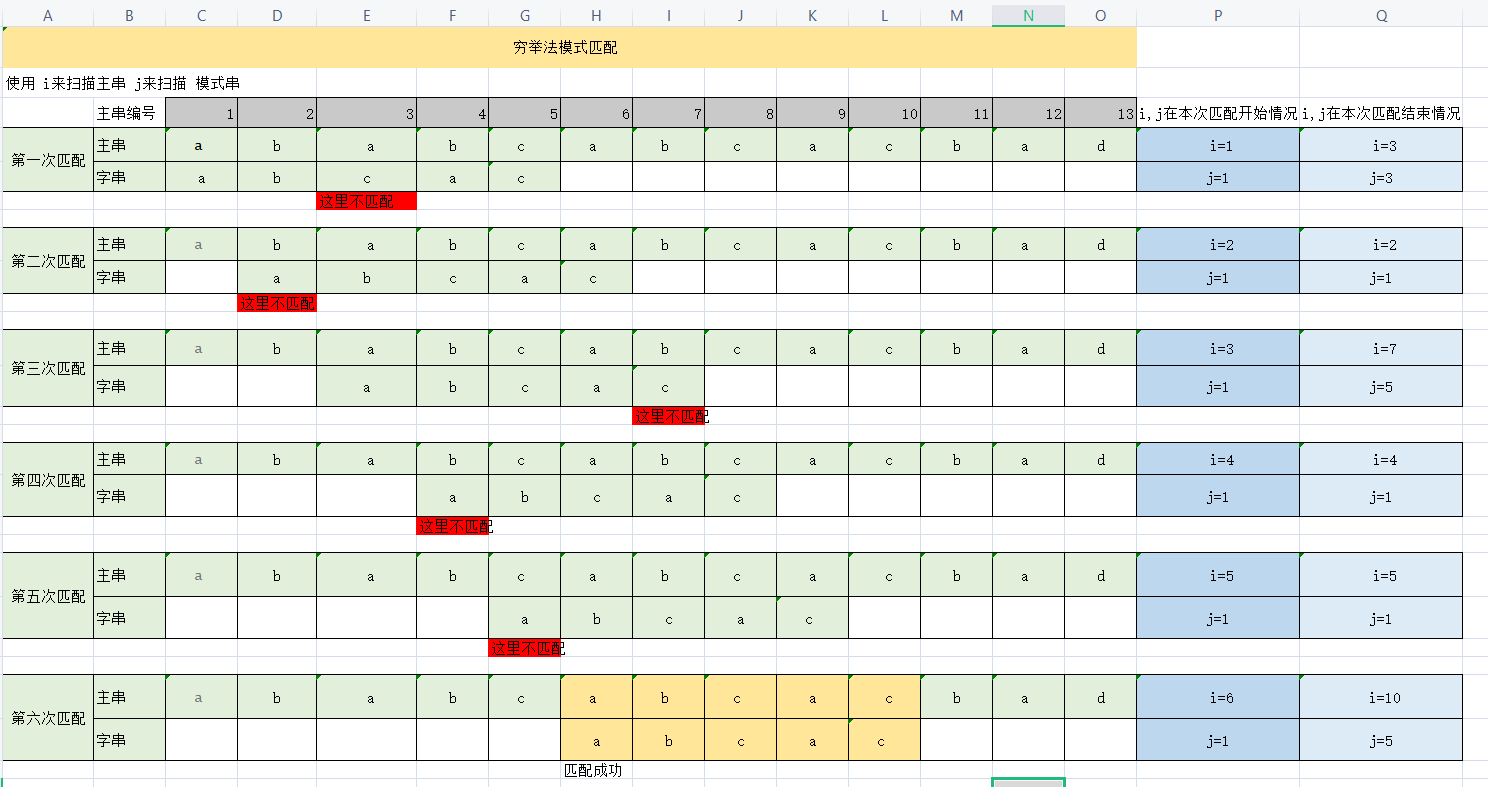int Index(SString S,SString T,int pos)
{
i=pos;j=1;
while(i<=S&&j<=T)
{
if(S[i++]==T[j++]);//如果相等 查看 下一个字符是否也匹配
else{i=i-j+2;j=1;} //i回溯到上一次取子串的下一个位置
}
if(j>T) return i-T;
else return 0;
}


while(i<=S&&j<=T) 这里是循环的出口 ，当i=S（S 存着 S串的长度）是 主串已经遍历完毕；j=T 说明子串遍历到了最后一个字符，说明匹配成功。

if(S[i++]==T[j++]); 这样看就简答多了 if(S[i]==T[j)i++;j++;

i=i-j+2; 这里就是 让i回到上一个位置， 讲起拆开来看，i=i-j+1 +1;

1. i-j+1 是让i回到 取子串的位置
2. 再+1 是为了向下一个遍历位置移动。

1. n-m+1 主串可以取得的子串个数
2. m 模式串多长 就意味着要对比多少次

## KMP 算法

KMP算法是对穷举法的优化，我们再穷举法里回溯 i，但是在 KMP算法中，我们可以不回溯i ？ 这是如何做到的呢？

### 人脑的思考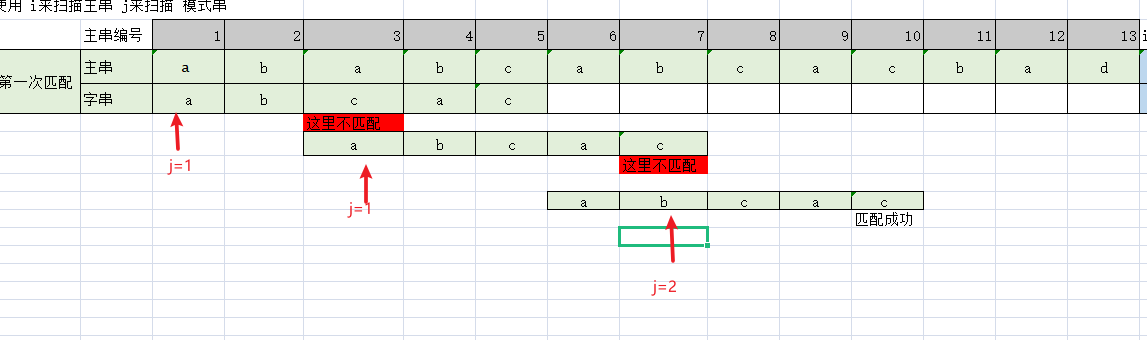1. 我们可以不回溯 i, 只回溯j，但是回溯j要做特殊处理
2. 模式串匹配的失败的地方，对于的j要回溯的地方和 主串无关
3. 模式串匹配失败的地方，前面的字符（或串）要和模式串的从第一个位置起（包含第一个字符）的串 一样。

1. 第一个位置匹配失败，当然j要回溯到 第一个位置 j=1

2. 第二个位置匹配失败 , j也只能回溯到第一个位置，如果回到第二个位置？不是刚匹配过吗？再对比有啥意义呢？ j=1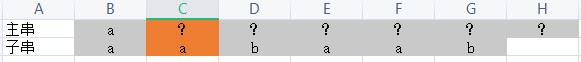3. 第三个位置匹配失败，说明此时匹配失败的地方？以前一个字符的字符一定是 a

所以j=2即可，检查此时的?是不是 a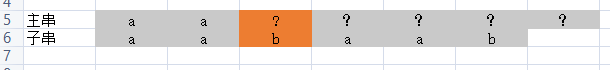4. 再第四个位置上匹配失败 ，说明 主串?之前的位置一定是b，此时j应该回溯到 j=1 检查？是不是a. 但是这里发现a和?匹配失败，说明此时 这个? 一定不是 a ，我们还需要 让 j=1 再去对比吗，不需要了。这里就说明本算法还有优化的空间，这我们后续再说！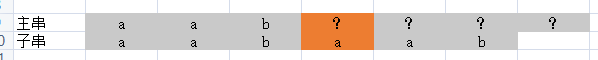5. j=2 ,和前面的推理一样

6. j=3，和前面的推理一样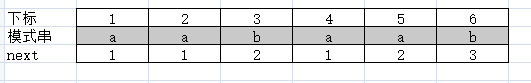### 数学证明

#### 使用结论口算 next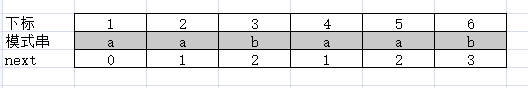### kmp 代码

int Index(SString S,SString T,int pos)
{
i=pos;j=1;
while(i<S&&j<T)
{
if(j==0||S[i]==T[i])
{
i++;j++;//开始对比下一个字符
}else j=next[j];// 回溯 j到 next[j] 位置上
}
if(j>T) return i-T;
else return 0;
}


if(j==0||S[i]==T[i]) 这里就得说明为啥 next=0

1. 当此时对比字符一样的时候，看看下一个字符是否一样
2. j回溯到模式串第一个字符的时候，并且主串与模式串第一个字符都不匹配
3. j回溯到模式串第一个字符的时候，并且主串与模式串第一个字符匹配

### 如何求得 next数组

next[j]=k ，就意味着，模式串j处字符前k个字符与模式串从第一个字符起，有k个字符一样且顺序相同。 求next[j+1]，我们其实在求解j+1处有多少字符和模式串从第一个字符起，字符一样且顺序相同。

next[j]=k说明， p1p2p....pk-1=pj-k+1p...pj-2pj-1 (前缀等于后缀)，这是我们前面推到处的公式哇p1p2p....pk=pj-k1+1p...pj-2pj-1 pj

### 代码实现

void get_next(SString T,int next[])
{
//next保存再 next中
i=1;next=0;
while(i<T)
{
if(j==0||T[i]==T[j])
{
i++;j++;next[i]=j;
}else
j=next[j];

}
}


1. i++;j++;

2. next[i]=j;

• 当i==j时 ，相当于 在执行前面推理的 next[j+1]=next[j]+1;
• 当i!=j时 ，相当于 在执行前面推理的 next[j+1]=next[k]+1;
1. j=next[j]; 这就是前面 取k1=next[k] 过程 ，即next[netx[j]]

## 优化next数组

### 代码实现

void get_next(SString T,int next[])
{
//next保存再 next中
i=1;next=0;
while(i<T)
{
if(j==0||T[i]==T[j])
{
i++;j++;
if(T[i]!=T[j]) next[i]=j;
else next[i]=next[j];
}else
j=next[j];
}
}


## 结语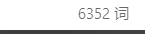Q.E.D.